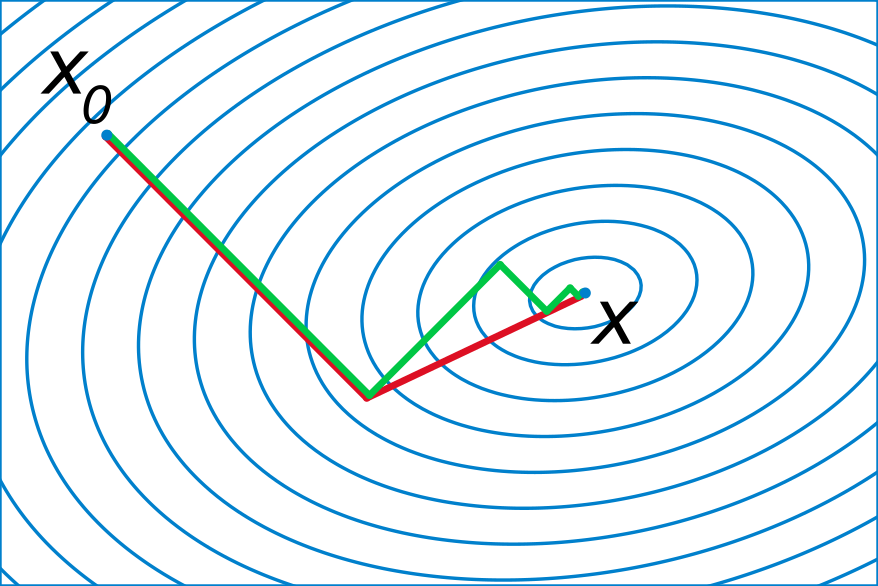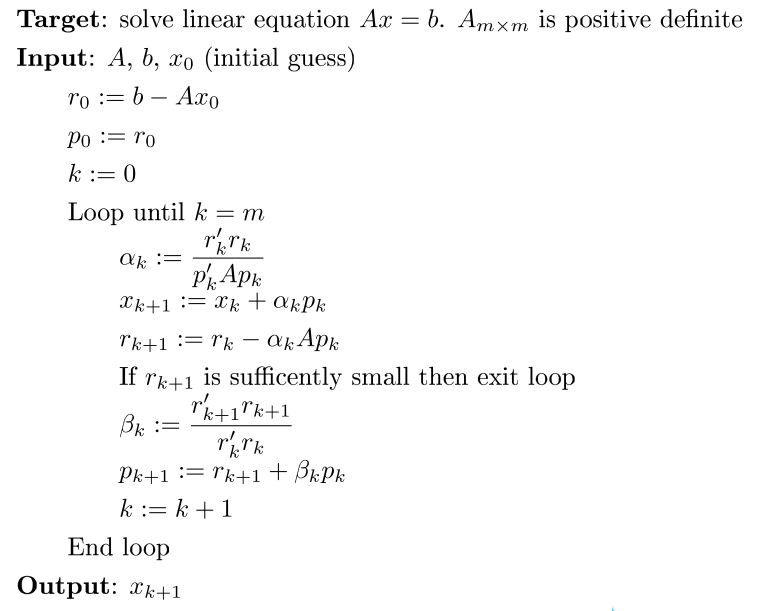# 什么是共轭梯度法？

$$x=\sum_{i=1}^m \alpha_i p_i.$$# 代码实现

## Target: solve linear equation Ax = b. A is positive definite
## Ax      -- A function to calculate the matrix-vector product
##            A * x given a vector x as the first argument
## b       -- Vector of the right hand side of the equation
## x0      -- Initial guess of the solution
## eps     -- Precision parameter
## verbose -- Whether to print out iteration information
cg = function(Ax, b, x0 = rep(0, length(b)), eps = 1e-6,
verbose = TRUE, ...)
{
m = length(b)
x = x0
r = b - Ax(x0, ...)
p = r
r2 = sum(r^2)
for(i in 1:m)
{
Ap = Ax(p, ...)
alpha = r2 / sum(p * Ap)
x = x + alpha * p
r = r - alpha * Ap
r2_new = sum(r^2)
err = sqrt(r2_new)

if(verbose)
cat(sprintf("Iteration %d, err = %.8f\n", i, err))

if(err < eps)
break
beta = r2_new / r2
p = r + beta * p
r2 = r2_new
}
x
}


## Simulation example
set.seed(123)
n = 10000
p = 1000
x = matrix(rnorm(n * p), n)
b = rnorm(p)
y = x %*% b

beta_direct = solve(crossprod(x), crossprod(x, y))

mat_vec_mult = function(x, mat)
{
as.numeric(crossprod(mat, mat %*% x))
}
xy = as.numeric(crossprod(x, y))
beta_cg = cg(mat_vec_mult, xy, mat = x)

max(abs(beta_direct - beta_cg))
##  7.422063e-12


Iteration 1, err = 80261.88521243
Iteration 2, err = 24276.83688338
...
Iteration 21, err = 0.00000622
Iteration 22, err = 0.00000197
Iteration 23, err = 0.00000062


# 真有这么神奇？

dat = read.csv("slice_localization_data.csv")
n = nrow(dat)
y = dat$reference x = as.matrix(dat[, -c(1, ncol(dat))]) / sqrt(n) xy = as.numeric(crossprod(x, y)) coeffs = cg(mat_vec_mult, xy, mat = x) ## Iteration 1, err = 11262.97730747 ## Iteration 2, err = 4471.54099614 ## Iteration 3, err = 1783.28640925 ## ... ## Iteration 100, err = 2.94723420 ## Iteration 101, err = 4.60232106 ## Iteration 102, err = 4.02014578 ## ... ## Iteration 200, err = 0.63018214 ## Iteration 201, err = 1.67568741 ## Iteration 202, err = 0.49243538 ## ... ## Iteration 382, err = 0.16954617 ## Iteration 383, err = 1.05050962 ## Iteration 384, err = 0.11322079  纳尼？？怎么跟剧本写的不一样啊？说好的提前收敛呢？就算不提前不是说最多 $m$ 步就收敛吗？我文章都写到这里了突然被打脸还怎么圆场啊？ （此处过去了半个小时……） 当崩塌的三观逐渐恢复的时候，就开始回过头来反思哪儿出了问题。其实，本文在之前有个非常重要的细节非常容易被忽视掉，大家把文章翻回第二节的第一句话，那里对矩阵 $A$ 加了一个定语：正定。正定的代数意义表现在矩阵所有的特征值都大于 0，而在回归中，它等价于数据矩阵 $X$ 是满秩的，换言之，没有多重共线性的存在。而如果我们检查一下这个数据中 $X'X$ 的行列式，就会发现它等于 0，也就是说有多重共线性的存在——原来我们之前兴冲冲地犯了一个美丽的错误。 知道哪儿出错了就好办了，对于多重共线性，其中的一种应对办法就是给 $X'X$ 的对角线上加上一个很小的常数 $\lambda$，这也就是我们常说的岭回归。我们重新修改一个岭回归版的矩阵运算函数，设定好 $\lambda\$ 参数和精度，再放到 CG 中去运行：

ridge = function(x, mat, lambda = 0.01)
{
as.numeric(crossprod(mat, mat %*% x)) + lambda * x
}
coeffs_ridge = cg(ridge, xy, eps = 1e-3, mat = x, lambda = 0.01)
## Iteration 1, err = 11256.55983300
## Iteration 2, err = 4455.13459864
## Iteration 3, err = 1767.78523995
## ...
## Iteration 61, err = 0.00164239
## Iteration 62, err = 0.00127173
## Iteration 63, err = 0.00092021


library(Matrix)
xsp = as(x, "sparseMatrix")
coeffs_sparse_ridge = cg(ridge, xy, eps = 1e-3, mat = xsp, lambda = 0.01)


# 总结

1. 实现简单，会矩阵乘法就行，不会的话请会的人吃顿饭就够了；
2. 内存占用小，妈妈再也不用担心花钱给我加内存了；
3. 可以控制收敛精度，想到哪儿停就到哪儿停；
4. 可以充分利用稀疏矩阵或者其他特殊的矩阵构造加快运算，激发小宇宙潜能。